## Data required for analysis

• One anatomical T1-weighted scan: anat.nii.gz
• One 4D fMRI task-related scan: fmri.nii.gz.
• Information on design:
• onsets/duration of stimuli
• Order of slices (which was first slice)
• or time slice measured (in ms).
• Repetition time (TR) from DICOM header/scanner/tech.

## Worked example: DICOM conversion

https://figshare.com/articles/SFO-example/5442298

url = paste0("https://ndownloader.figshare.com/articles/",
"5442298/versions/1")
zipfile = tempfile(fileext = ".zip")
res = httr::GET(url, write_disk(path = zipfile))

####### unzip file code (not shown) ###########

out_files = c("anat.nii.gz", "fmri.nii.gz")

## fMRI information

fmri_filename = "fmri.nii.gz"
tr = 1.8 # seconds
(nslices = oro.nifti::nsli(hdr))
 60
(n_time_points = oro.nifti::ntim(hdr))
 280
hdr
NIfTI-1 format
Type            : nifti
Data Type       : 16 (FLOAT32)
Bits per Pixel  : 32
Slice Code      : 0 (Unknown)
Intent Code     : 0 (None)
Qform Code      : 1 (Scanner_Anat)
Sform Code      : 2 (Aligned_Anat)
Dimension       : 96 x 96 x 60 x 280
Pixel Dimension : 2.25 x 2.25 x 2.55 x 1.8
Voxel Units     : mm
Time Units      : sec

## Explore the Data

The first part of any preprocessing pipeline should be to use exploratory techniques to investigate detect possible problems and artifacts.

fMRI data often contain transient spike artifacts and slow drift.

An exploratory technique such as principal components analysis (PCA) can be used.

(courtesy of Martin Lindquist)

## Types of Registration

• Rigid-body registration (linear) - 6 degrees of freedom (dof)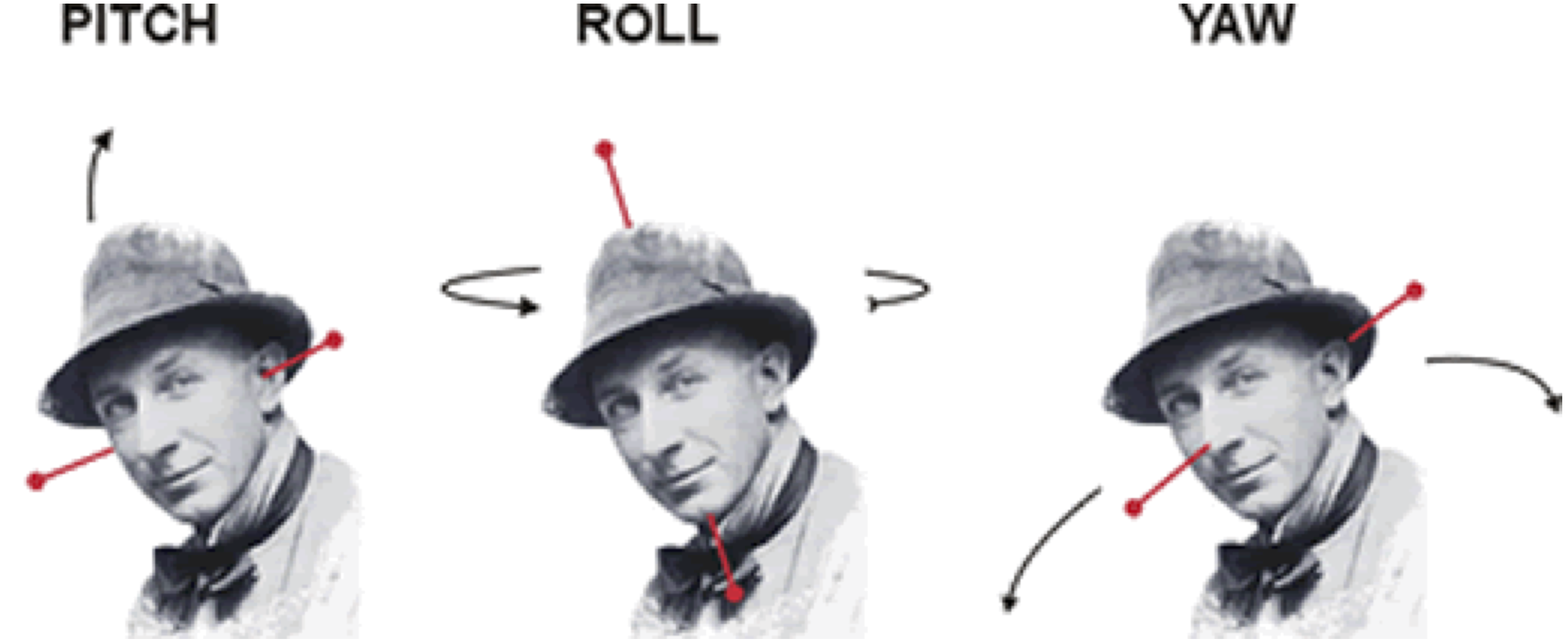Image taken from http://cnl.web.arizona.edu/imageprops.htm

• Pitch - Think of nodding (“yes”)
• Yaw - Think of shaking head (“no”)
• Roll - Think of shoulder shrugging (“I don’t know”)
• x – left/right, y – forward/backward, z – jump up/down

## Rigid Registration: The Math

For a voxel $$v$$, the rigid transformation can be written as:

$T_{\rm rigid}(v) = Rv + t$ where $$R =$$ $\left[\begin{array}{ccc} \cos\beta\cos\gamma& \cos\alpha\sin\gamma + \sin\alpha\sin\beta\cos\gamma & \sin\alpha\sin\gamma - \cos\alpha\sin\beta\cos\gamma \\ -\cos\beta\sin\gamma & \cos\alpha\cos\gamma - \sin\alpha\sin\beta\sin\gamma & \sin\alpha\cos\gamma + \cos\alpha\sin\beta\sin\gamma \\ \sin\beta & -\sin\alpha\cos\beta & \cos\alpha\cos\beta \end{array}\right]$

• 6 degrees of freedom
• $$3$$ associated with the translation vector: $$t=(t_x, t_y, t_z)$$
• $$3$$ associated with the rotation parameters: $$\theta=(\alpha, \beta,\gamma)$$.

## Image Realignment: within-fMRI registration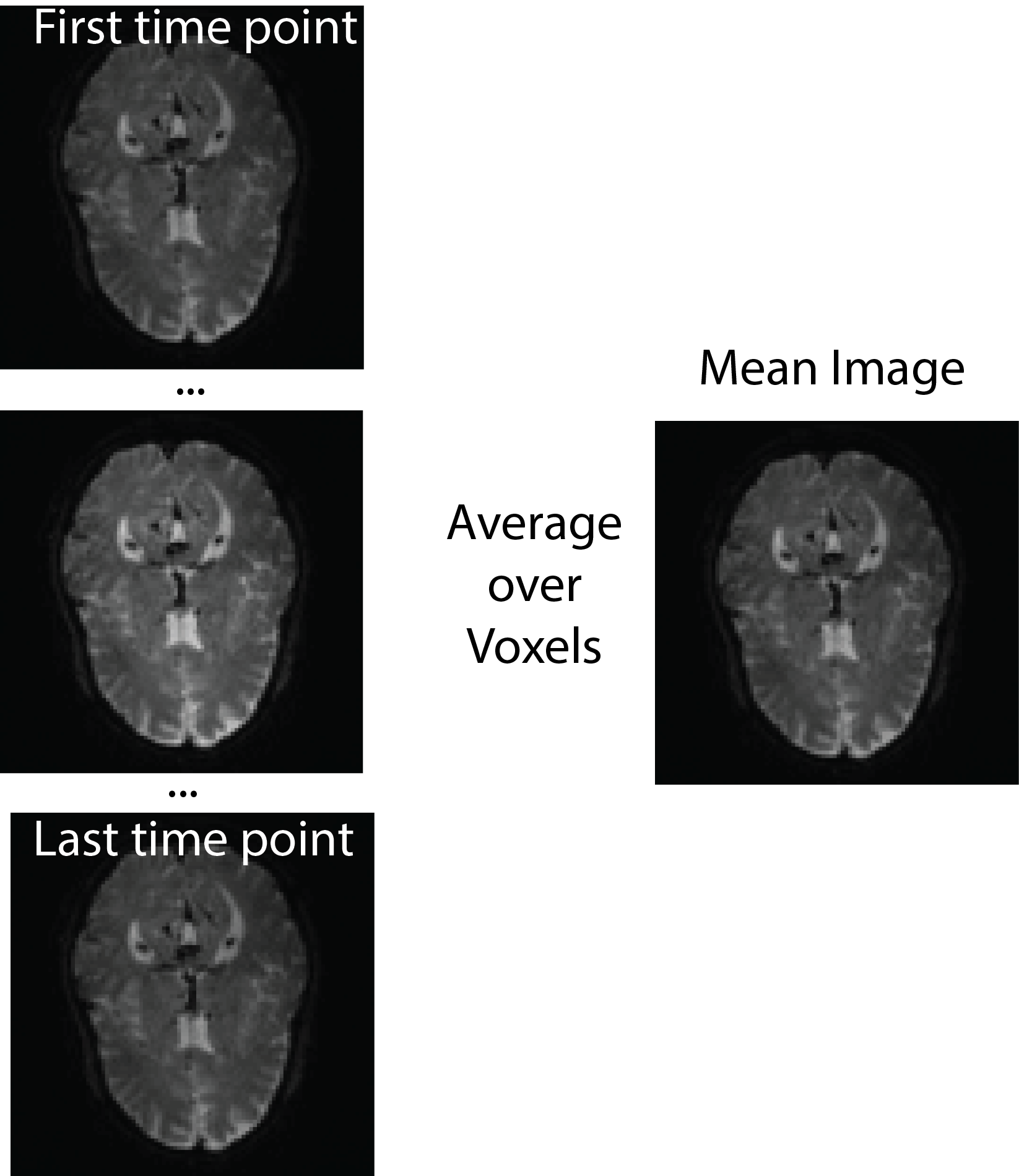## Image Realignment

realigned = spm12_realign(filename = fmri_filename,
time_points = seq(n_time_points),
quality = 0.98, separation = 3,
register_to = "mean", est_interp = "bspline4", reslice_interp = "bspline4")
# reading in the mean image
mean_img = realigned[["mean"]]
realignedoutfiles  "rfmri.nii" realignedmat
 "fmri.mat"

## Image Realignment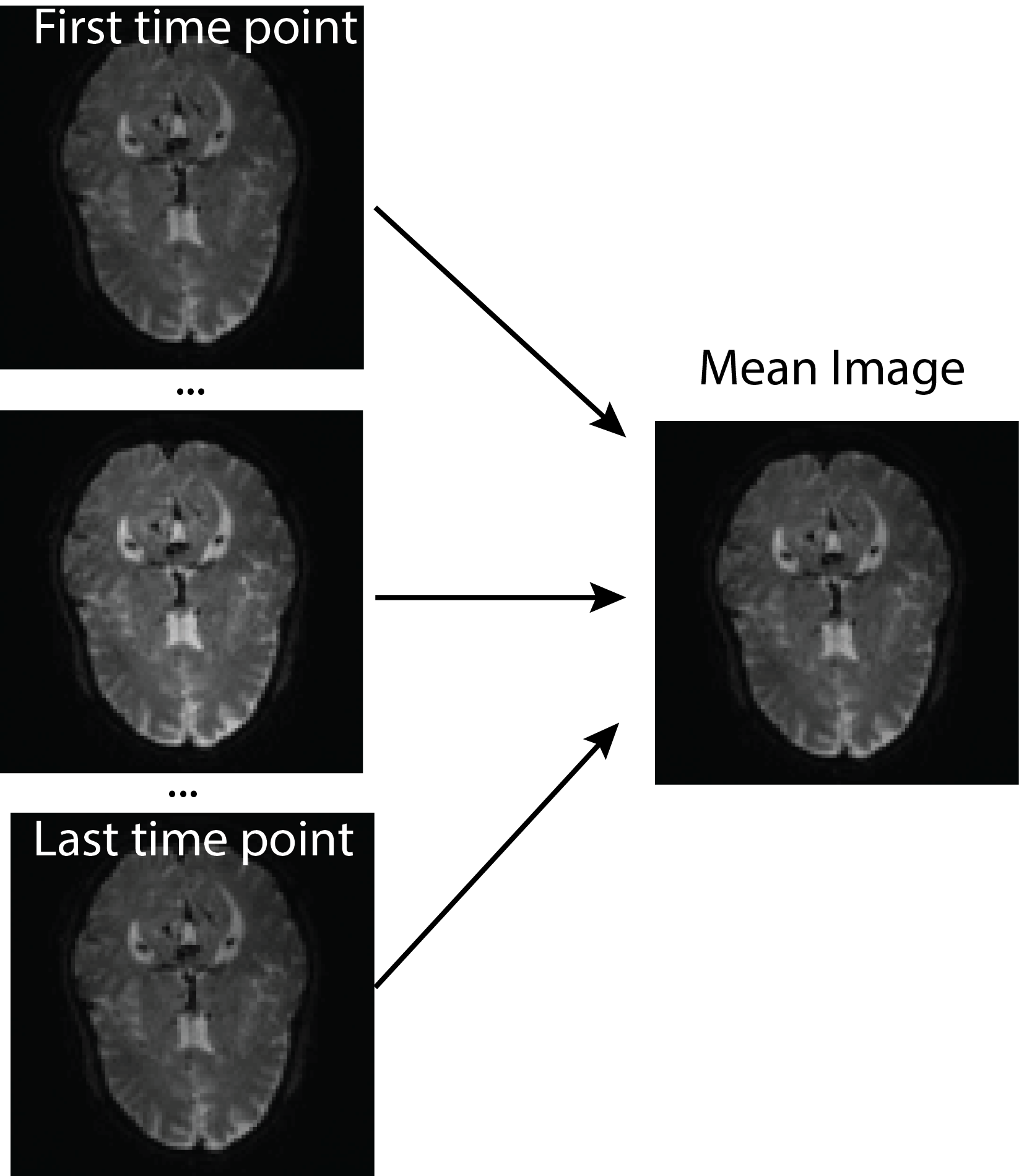## Plotting the realignment parameters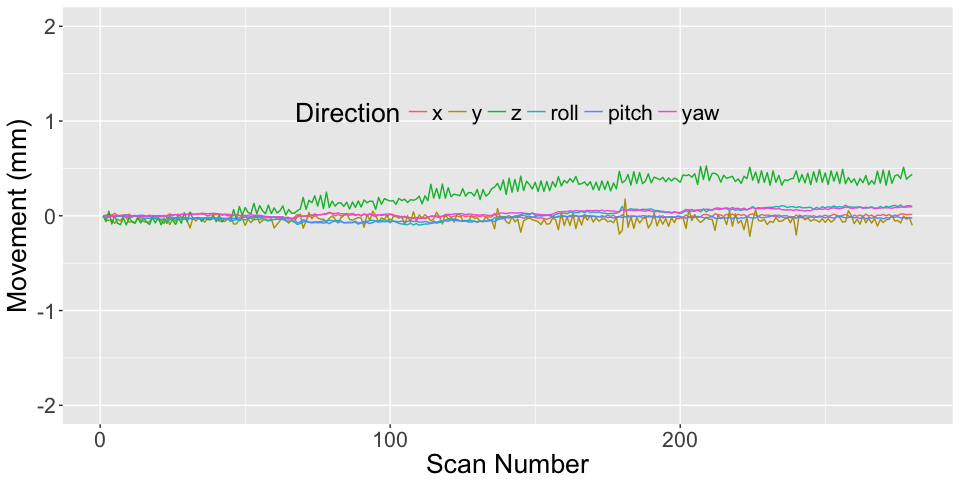## Slice timing correction - temporal alignment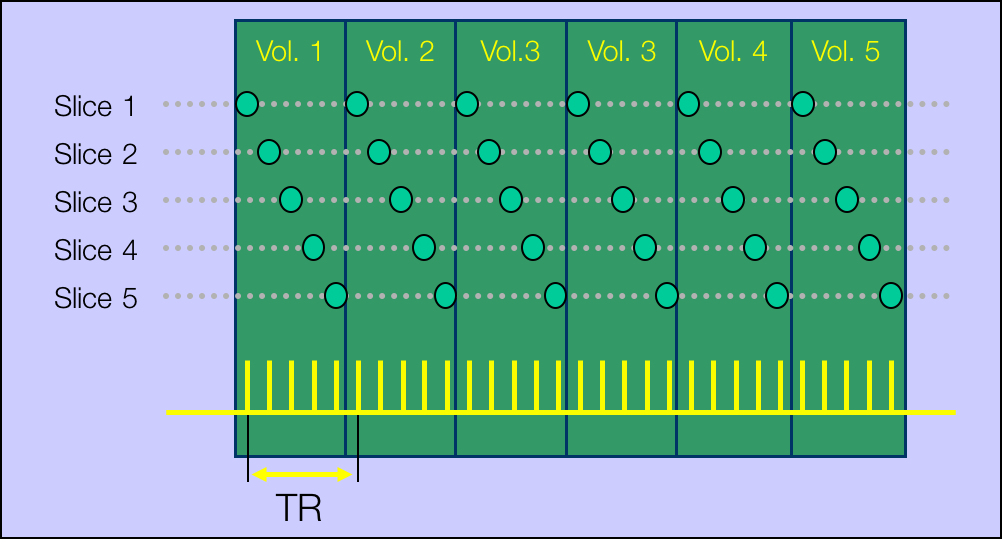(courtesy of Martin Lindquist)

## Slice timing correction - temporal alignment

• slice order: descending, dual-coil (different for ascending or interleaved)
• Need to know this from DICOM/design
slice_order = c(1740, 1680, 1620, 1560, 1500, 1440, 1380,
1320, 1260, 1200, 1140, 1080, 1020, 960, 900, 840, 780,
720, 660, 600, 540, 480, 420, 360, 300, 240, 180, 120, 60,
0, 1740, 1680, 1620, 1560, 1500, 1440, 1380, 1320, 1260,
1200, 1140, 1080, 1020, 960, 900, 840, 780, 720, 660, 600,
540, 480, 420, 360, 300, 240, 180, 120, 60, 0)
ref_slice = 900
ta = 0 # since slice_order in ms

## Data needed for slice timing correction

• Repetition time (from hdr)
• Number of time points and slices (from hdr)
• Slice order + need the reference slice (ref_slice),
• Time between the first and the last slice within one scan (ta). ta = 0 if you give slice order in seconds/milliseconds.

## Slice timing correction - temporal alignment

aimg = spm12_slice_timing(filename = realigned$outfiles, nslices = nslices, tr = tr, slice_order = slice_order, time_points = seq(n_time_points), ta = ta, # since slice order given in ms ref_slice = ref_slice, prefix = "a") print(aimg$outfile)

## After lice timing correction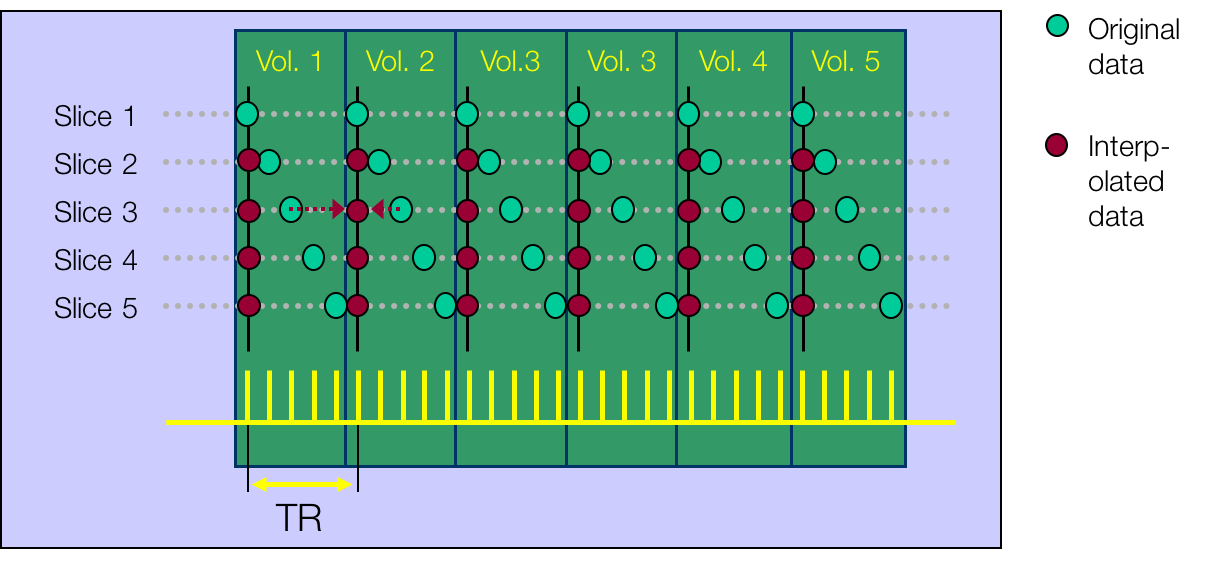(courtesy of Martin Lindquist)

## T1 Coregistration to Mean fMRI

We then perform the coregistration of the mean image (fixed) and T1 (moving):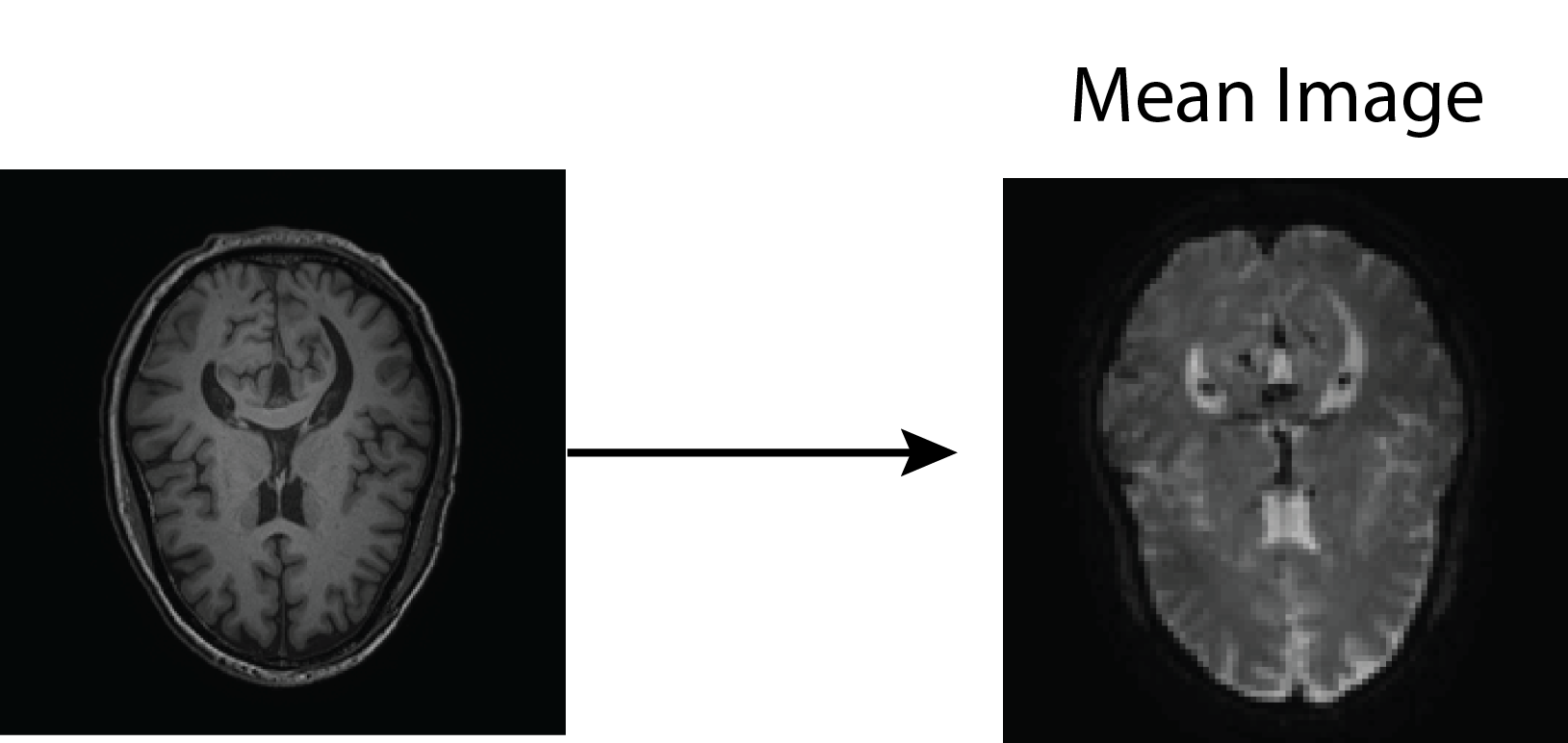## T1 Coregistration to Mean fMRI

Coregistration is estimated using spm12_coregister_estimate:

t1_fname = "anat.nii.gz"
coreg = spm12_coregister_estimate(
fixed = mean_img,
moving = t1_fname,
cost_fun = "nmi")
coreg$outfile  "anat.nii" ## Output file was the same: nothing happened! • spm12_coregister_estimate - estimates coregistration (transforms the header) • spm12_coregister_reslice - reslices the image to the same voxel dimensions (should probably be coregistered already using estimate) • spm12_coregister - estimates and reslices • Estimate the transformation, but do segmentation on native T1 space (better resolution) ## Anatomical MRI Segmentation Here we segment the image into 6 different regions, where the regions are gray matter, white matter, cerebrospinal fluid (CSF), bone, soft tissue, and the background. seg = spm12_segment( filename = coreg$outfile,
set_origin = FALSE,
bias_corrected = TRUE, native = TRUE,
unmodulated = TRUE, modulated = TRUE, affine = "mni",
sampling_distance = 1.5)

## Anatomical MRI Segmentation

• native - native space segmentations
• modulated - adjusted segmentations to constrain tissue-class volumes
• unmodulated - unadjusted
• bias_corrected - save bias-field corrected image
• set_origin - should AC/PC alignment be done (no because we just coregistered)

## Anatomical MRI Segmentation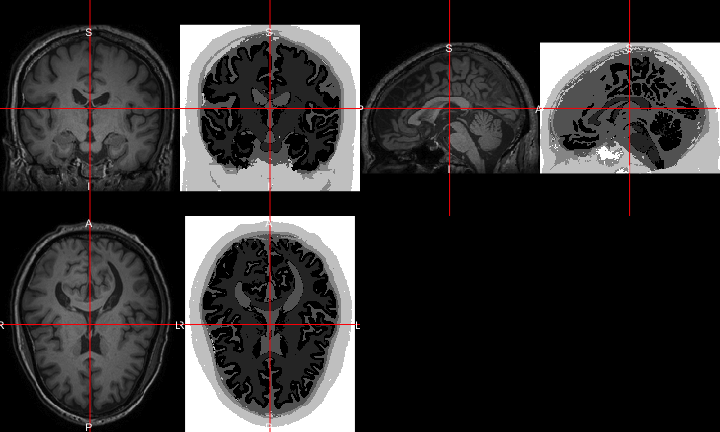## Anatomical MRI Segmentation: CSF/WM/GM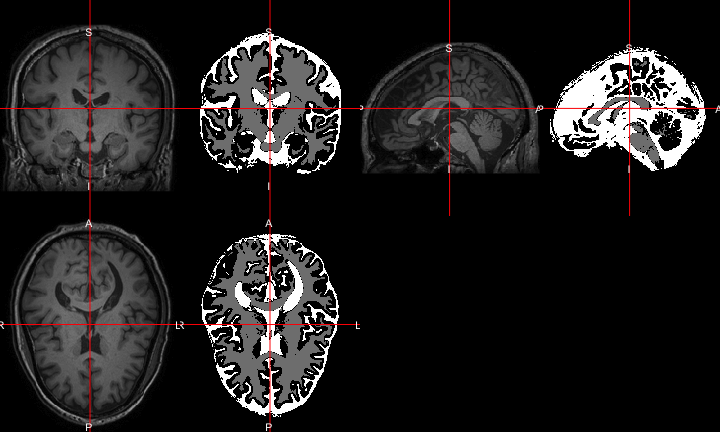## Spatial normalization to MNI

• My brain is not the same size/shape as your brain
• Want to look across subjects spatially
• Spatial normalization allows us to transform the data, stretching and scaling the data (nonlinearly) to a standard brain.
• MNI (Montreal Neurological Institute) is the most commonly used (ICBM MNI152 of some sort, http://www.bic.mni.mcgill.ca/ServicesAtlases/ICBM152NLin2009).

## Spatial normalization to MNI

Affine + Non-linear transform (invertible)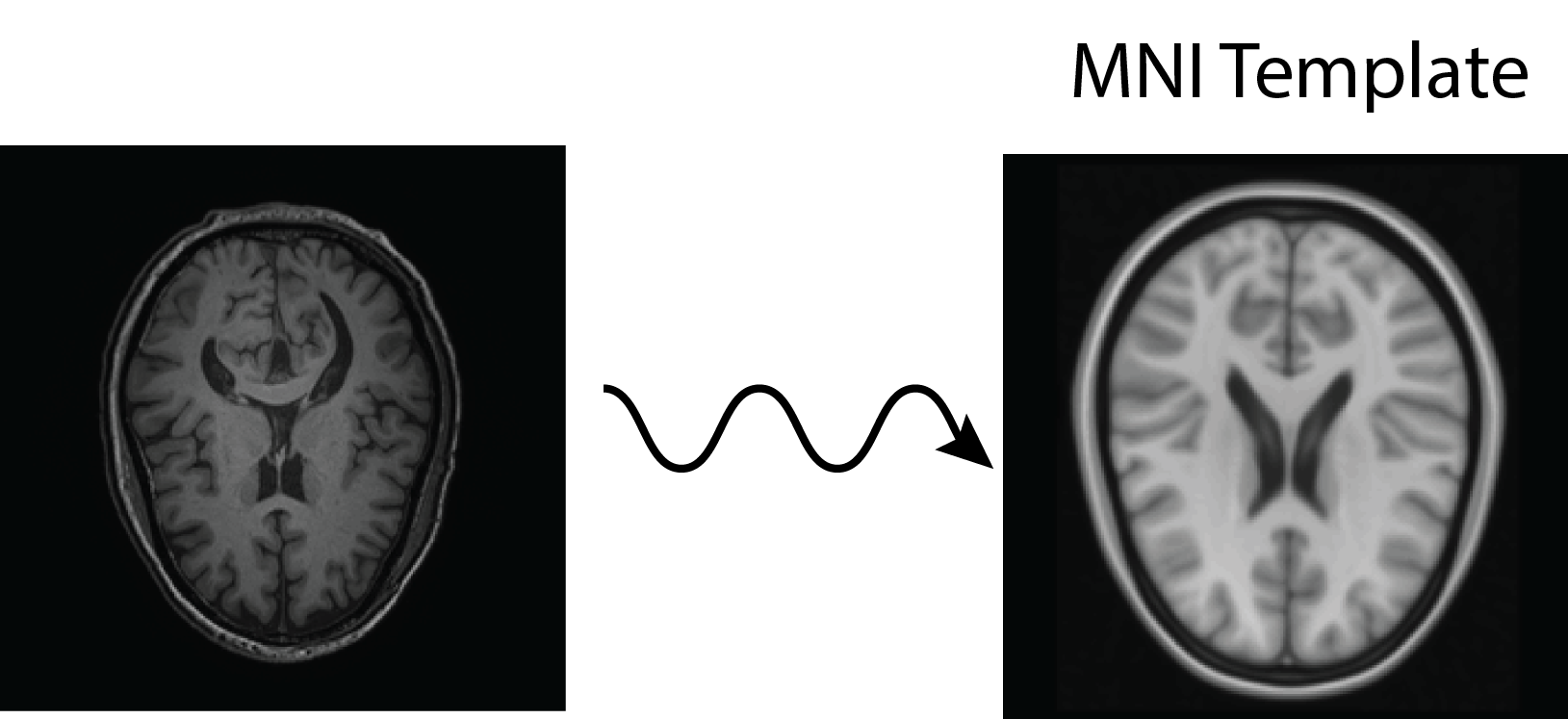## Spatial normalization to MNI: already done

The segmentation was done by warping the T1 to the MNI template and that transform/deformation in the segmentation output:

other.files = aimg$outfile, #corrected fMRI bounding_box = bounding_box, interp = "bspline5") ## Applying spatial normalization: fMRI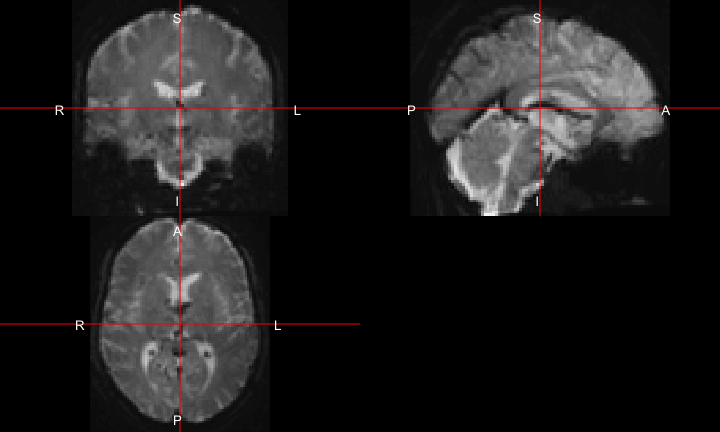## Applying spatial normalization: Corrected T1 anat_norm = spm12_normalize_write(deformation = seg$deformation,
other.files = seg$bias_corrected, bounding_box = bounding_box, interp = "bspline5", voxel_size = c(1, 1, 1)) anat_norm$outfiles
 "wmanat.nii"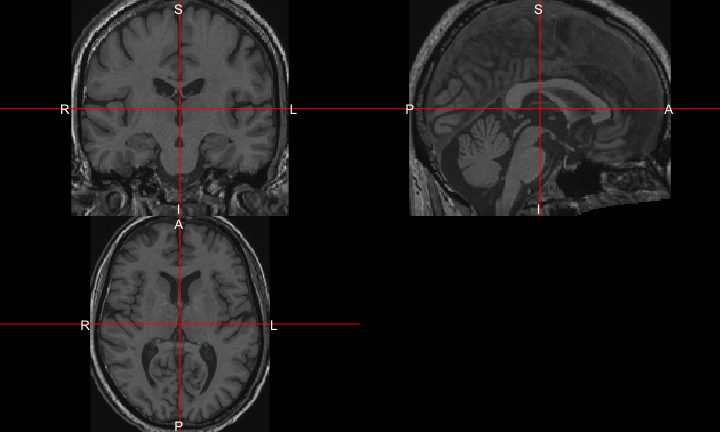## Applying spatial normalization: T1, but 2x2x2

anat_norm2x2x2 = spm12_normalize_write( deformation = seg$deformation, other.files = seg$bias_corrected, bounding_box = bounding_box,
interp = "bspline5", voxel_size = c(2, 2, 2)) # note the resolution!!!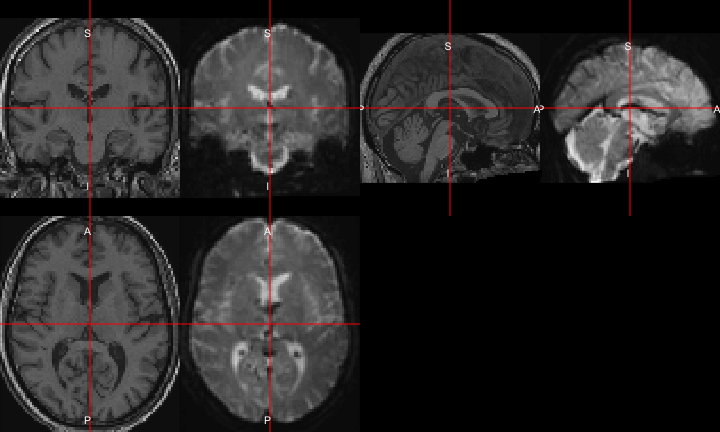## Spatial smoothing using a Gaussian

• Spatial smoothing should signal to noise depending on the size of activation

• Typically, the amount of smoothing is chosen a priori

• Usually global smoothing (same amount at each voxel), but can be adaptive (adimpro pacakge)

• Specified using the full-width half max (FWHM) for the Gaussian smoother (not $$\sigma$$): $$FWHM = \sigma \sqrt{8 \log(2)}$$

## Spatial smoothing using a Gaussian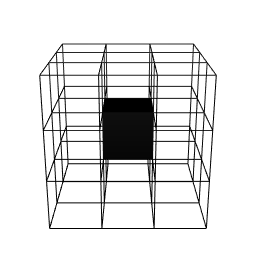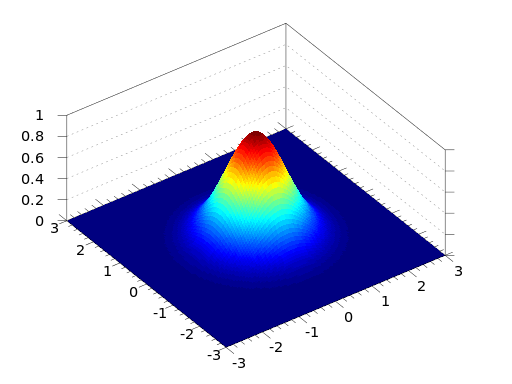## Spatial smoothing using a Gaussian

smooth_norm = spm12_smooth(
normoutfiles[], fwhm = 5, prefix = "s5")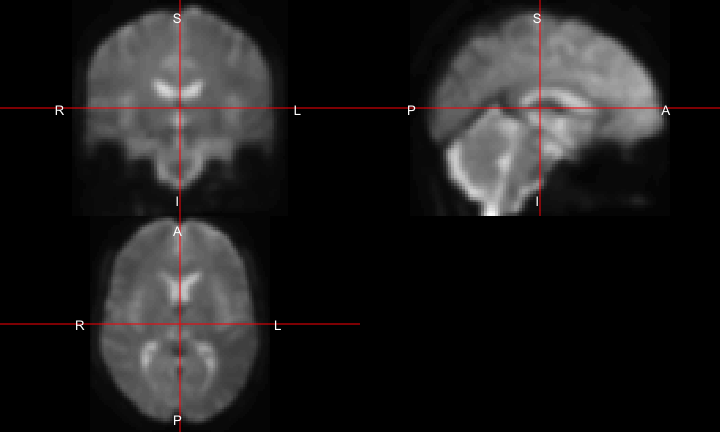## First level modeling: Single-subject model In many applications, that smoothed data you will use for post-processing and analysis. Motion correction has usually been applied above, but some realign this again. ## Conditions of the experiment (block design) • need the onset/duration of conditions (in seconds or scans): condition_list = list( list(name = "LeftHand", onset = c(20, 100, 180, 260, 340, 420), duration = c(20, 20, 20, 20, 20, 20) ), list(name = "RightHand", onset = c(60, 140, 220, 300, 380, 460), duration = c(20, 20, 20, 20, 20, 20) ) ) ## First level modeling: single-subject model • Conditions are convolved with the Hemodynamic Response Function (HRF)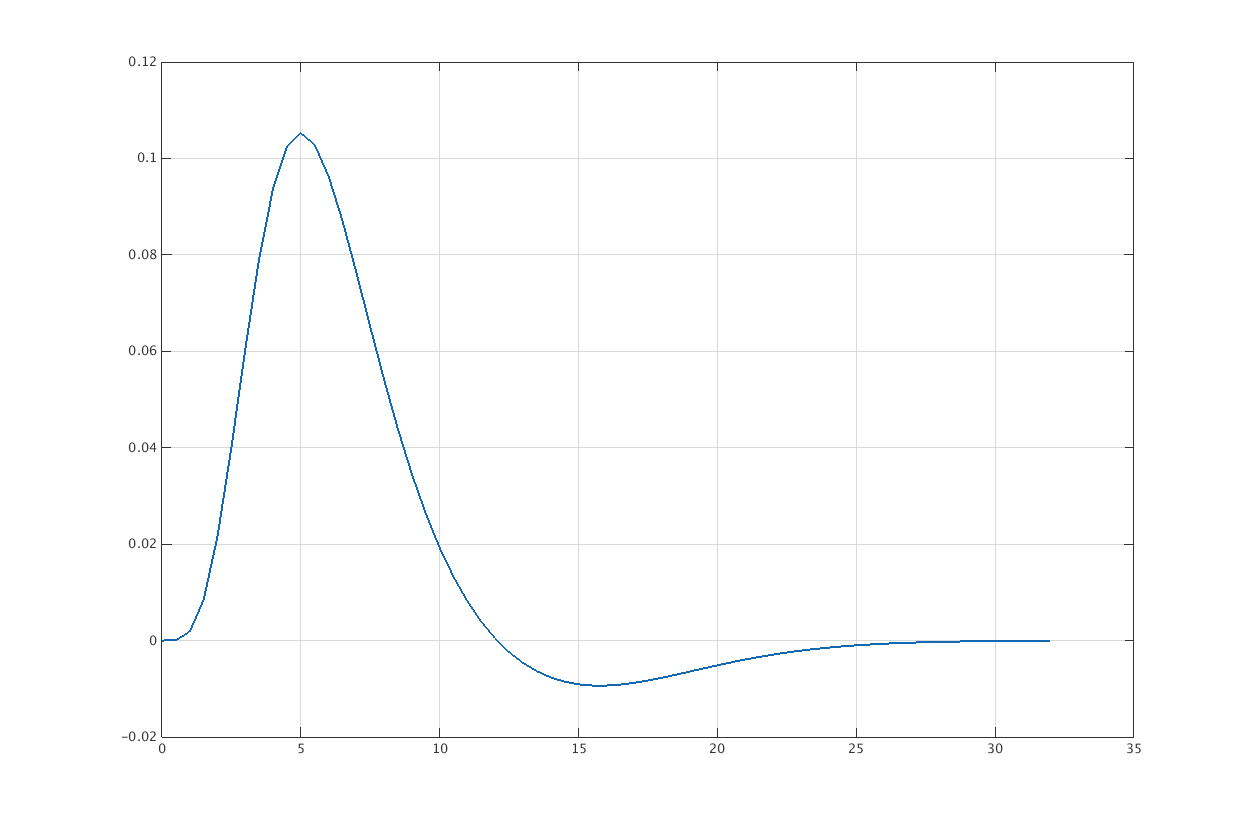## Estimate first level model • General linear model (GLM) (not Generalized) • regressor_mat - motion parameters and other “confounders” (not convolved with HRF) • condition_list - conditions are convolved first_model = spm12_first_level( scans = smooth_normoutfiles,
n_time_points = n_time_points,
units = "secs", slice_timed = TRUE,  tr = tr,
condition_list = condition_list, regressor_mat = rpfile)

## Model outputs: Cheat Sheet

• beta coefficient maps of regressors and contrasts
betas = list.files(pattern = "beta.*[.]nii"); print(betas)
 "beta_0001.nii" "beta_0002.nii" "beta_0003.nii" "beta_0004.nii"
 "beta_0005.nii" "beta_0006.nii" "beta_0007.nii" "beta_0008.nii"
 "beta_0009.nii"
• SPM.mat - model specification
print(first_model$spmmat)  "SPM.mat" ## Contrast Manager - Creating Contrasts • can make T-statistic of F statistic maps • weights indicate which coefficients contrasts = list( list(name = "LeftHand", weights = c(1, rep(0, 7)), replicate = "none", type = "T" ), list(name = "RightHand", weights = c(0, 1, rep(0, 6)), replicate = "none", type = "T"), list(name = "AllEffects", weights = rbind( c(1, rep(0, 7)), c(0, 1, rep(0, 6)) ), replicate = "none", type = "F") ) ## Contrast Manager - Creating Contrasts contrast_res = spm12_contrast_manager(spm = first_model$spmmat,
delete_existing = TRUE, contrast_list = contrasts)
cons = list.files(pattern = "con.*[.]nii")
print(cons)
 "con_0001.nii" "con_0002.nii"
stats = list.files(pattern = "spm(T|F).*[.]nii")
print(stats)
 "spmF_0003.nii" "spmT_0001.nii" "spmT_0002.nii"

## Displaying contrasts: contrast 1 (LeftHand)

spmt = readnii("spmT_0001.nii")
ortho2(norm, spmt)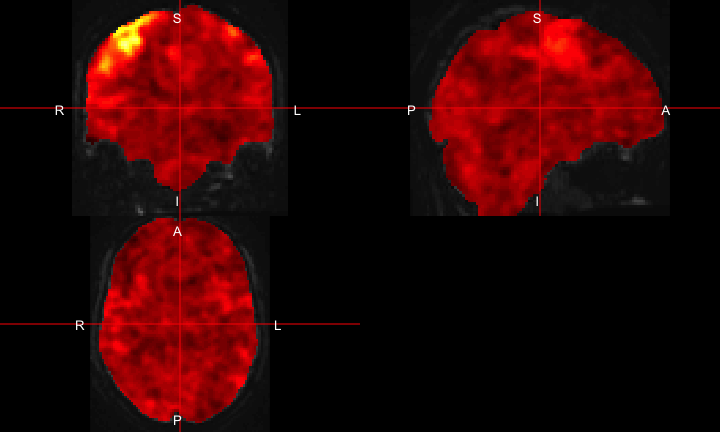## Displaying Contrasts wheere T > 5

ortho2(norm, spmt > 5)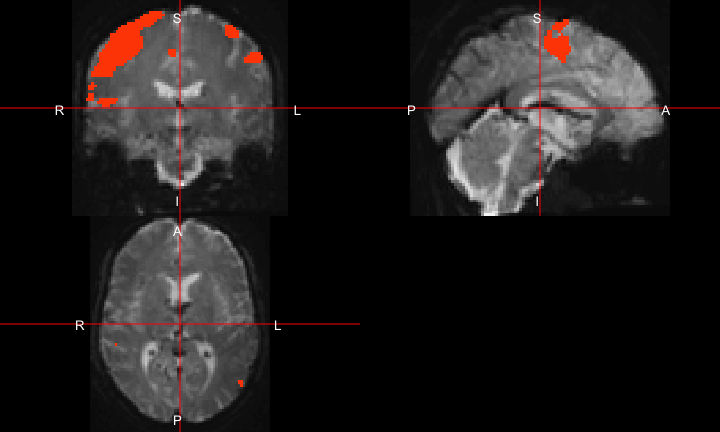## There is no universal fMRI pipeline

• Each step has inherent drawback and limitation (spatial resolution, artifact smoothing, etc.)
• A few different pipelines should be tested.
• Not necessarily all combinations, but change the “knobs” a bit
• Similar to sensitivity analysis

## Why spm12r

• Can integrate into your R pipeline
• May be helpful for developing new methods/simulations/testing
• More advanced statistical methods in R may be available
• If you know R you’re good